# RSoft Application Gallery Note: Single- and Multi-Stage Ring Resonators

## Tool Used: OptSim Circuit

Ring resonator is an important component in silicon photonics. It is made up of an optical waveguide in a loop fashion with coupling mechanism as shown in the schematic of Figure 1. The mode-locked laser acts as a wideband source and has an extremely narrow pulse-width. As per the theory of ring resonators , the ring is in resonance when wavelength of the light fits integer number of times in the optical length of the ring. The resonance wavelength is given by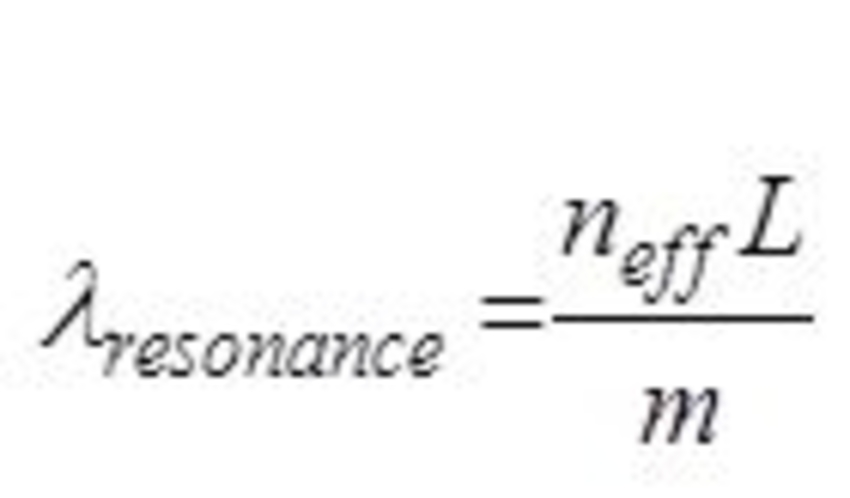where m is a non-zero positive integer, L is the optical roundtrip length of the ring, and neff is the effective index of the waveguide.

Free spectral range (FSR) is an important specification of a ring resonator. It is the spacing between resonances and is given by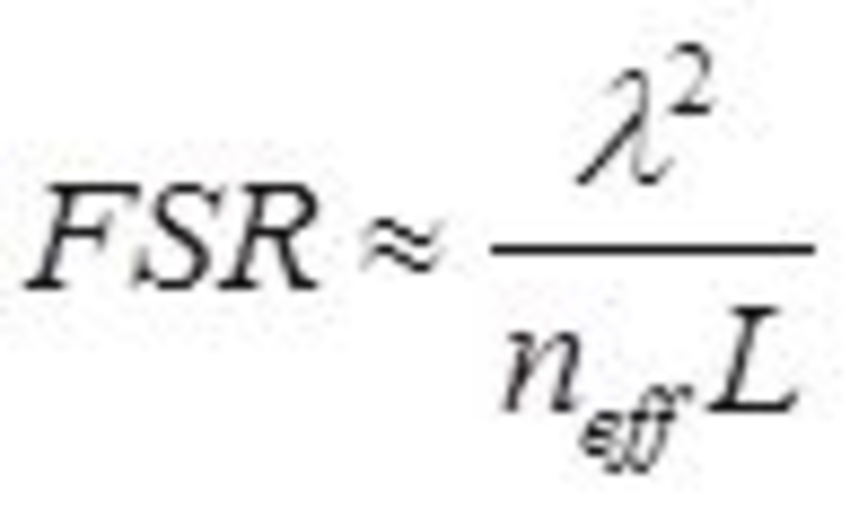The group refractive index can be used in above expression instead of the effective index for more accurate values.

Another parameter often specified is finesse of a ring resonator filter which is a ratio of FSR to full-width-half-maximum (FWHM) of a resonance for a specific wavelength.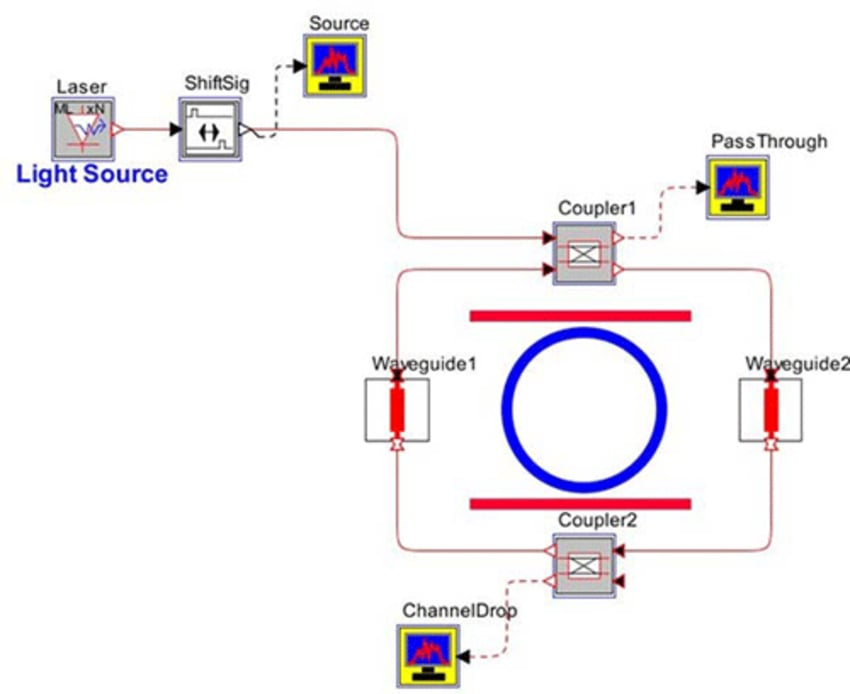Figure 1: Layout of a single-stage ring resonator circuit

The pass-through and drop spectra are shown below in Figures 2 and 3, respectively.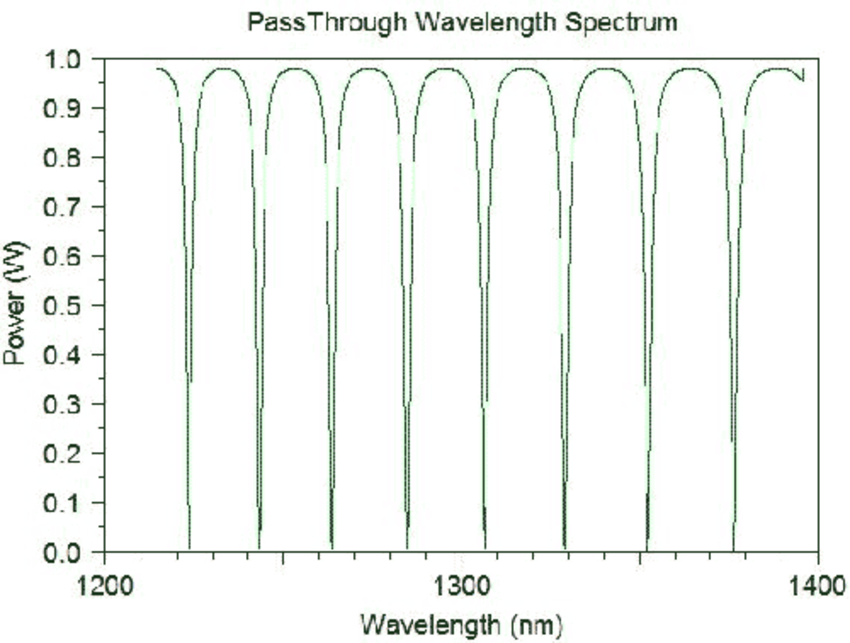Figure 2: Pass-through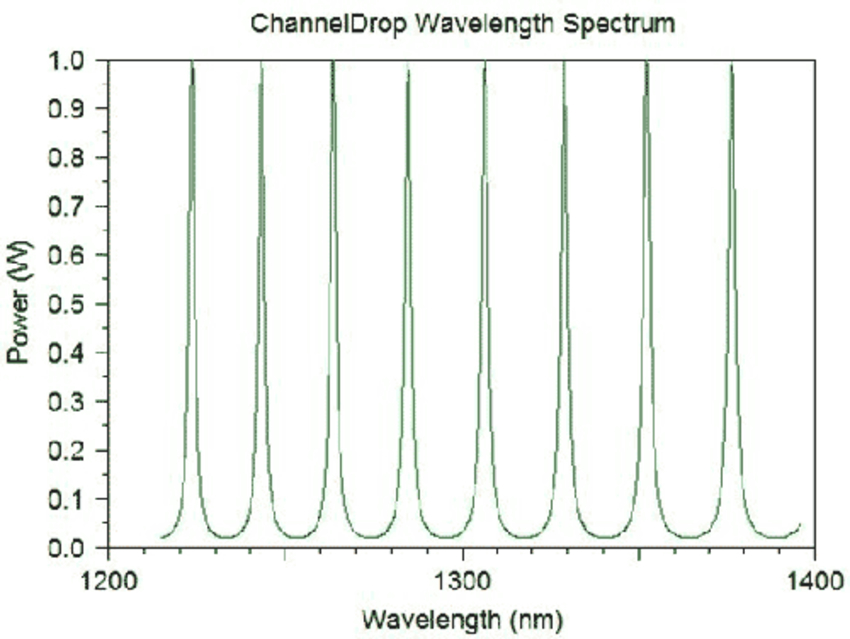Figure 3: Drop spectrum

As shown in Fig. 4, the measured value of the FSR matches with the analytically evaluated value.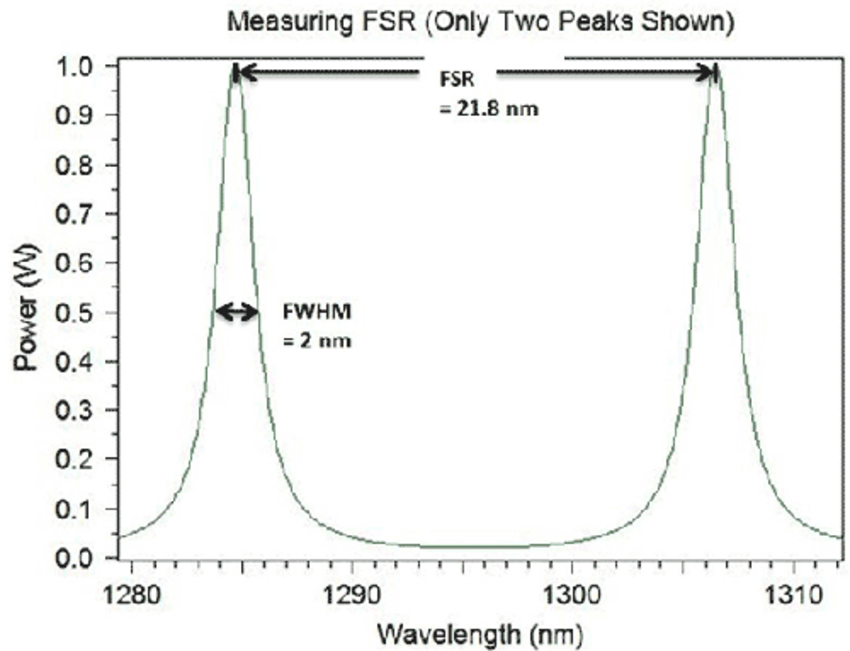Figure 4: FSR and FWHM measurements

The ratio of FSR to full-width half-maximum (FWHM) is the Finesse, which for the above case is 10.9. The quality factor (Q-factor) is a measure of sharpness of the resonance relative to its central frequency (ratio of resonance frequency to FWHM), which for the current setup is around 650.

OptSim Circuit allows modeling of PICs that are cascaded (or arranged as multi-stage). For example, the single-stage ring resonator PIC that we used in the discussion above can be cascaded as shown in Fig. 5.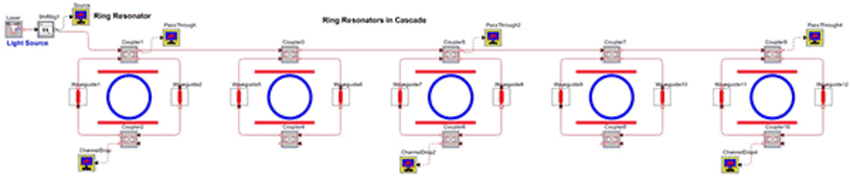Figure 5: Cascaded stages of ring resonators

Figure 6 shows pass through spectrum of the last stage of the PIC.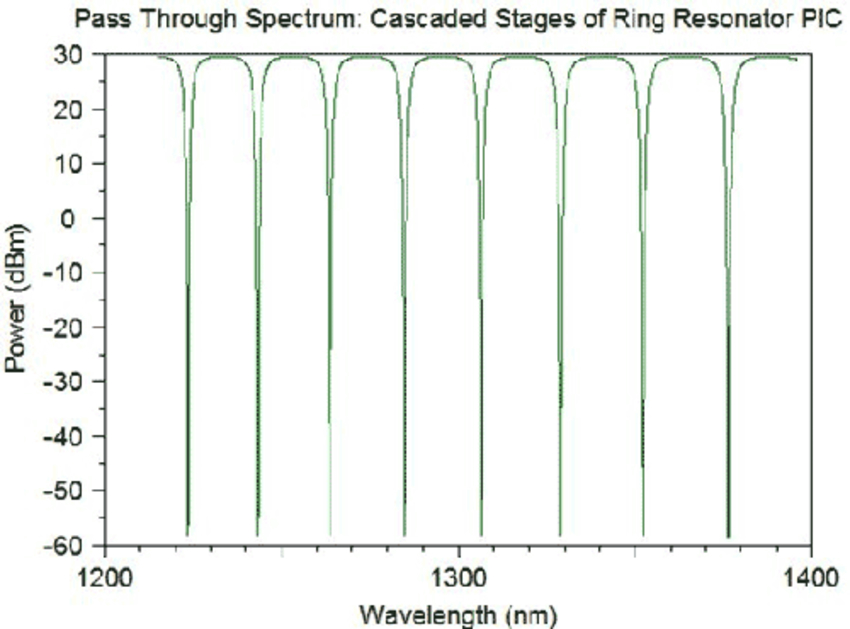Figure 6: Pass through spectrum of the multi-stage ring resonator PIC

## References:

1 W. Bogaerts, et al., "Silicon mircroring resonators," Laser Photonics Review, vol. 6, no. 1, pp. 47-53, 2012.